Rev.2.00 Feb 07, 2007 page 1 of 23
HA1630D04/05/06 Series
Ultra-Small Low Voltage Operation CMOS Dual Operational
Amplifier REJ03D0801-0200
Rev.2.00
Feb 07, 2007
Description
The HA1630D04/05/06 are high slew rate dual CMOS Operational Amplifiers realizing low voltage operation, low
input offset voltage and low supp ly current. In addition to a low operating voltag e from 1.8V, these device output can
achieve full swing output voltage capability extending to either supply. Available in an ultra-small TSSOP-8 and
MMPAK-8 pa ckage that occupy more small area against the SOP-8.
Features
Low power and single supply operation VDD = 1.8 to 5.5 V
Low input offset voltage VIO = 4.0 mV Max
Low supply current (per channel) IDD = 200 μA Typ (HA1630D04)
I
DD = 400 μA Typ (HA1630D05)
I
DD = 800 μA Typ (HA1630D06)
High slew rate SR = 2 V/μs Typ (HA1630D04)
SR = 4 V/μs Typ (HA1630D05)
SR = 8 V/μs Typ (HA1630D06)
Maximum output voltage VOH = 2.9 V Min (at VDD = 3.0 V)
Low input bias current IIB = 1 pA Typ
Ordering Information
Type No. Package Name Package Code
HA1630D04T
HA1630D05T
HA1630D06T TTP-8DA PTSP0008JC-B
HA1630D04MM
HA1630D05MM
HA1630D06MM MMPAK-8 PLSP0008JC-A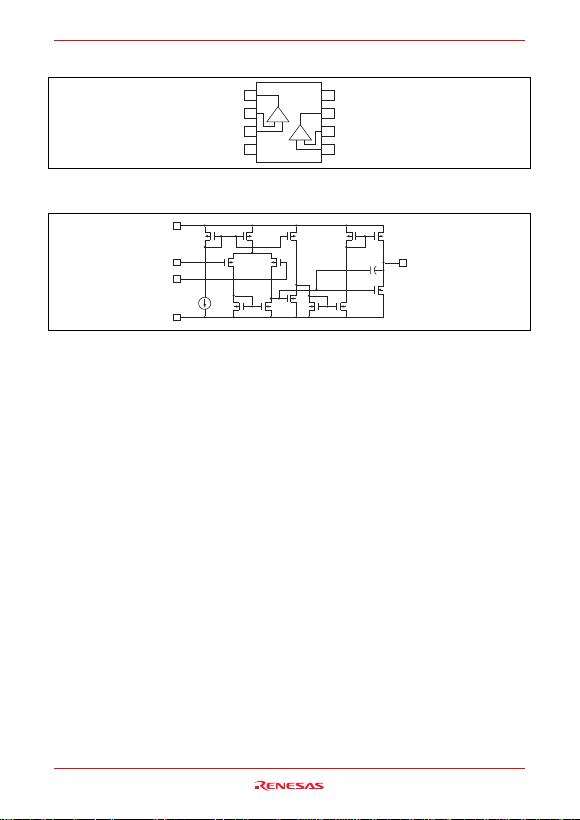HA1630D04/05/06 Series
Rev.2.00 Feb 07, 2007 page 2 of 23
Pin Arrangement
VDD
VOUT2
VIN2(–)
VIN2(+)
VOUT1
VIN1(–)
VIN1(+)
VSS
8
7
5
6
1
2
4
3
+
+
Equivalent Circuit (per one channel)
VDD
VSS
VIN(+)
VIN(–) VOUT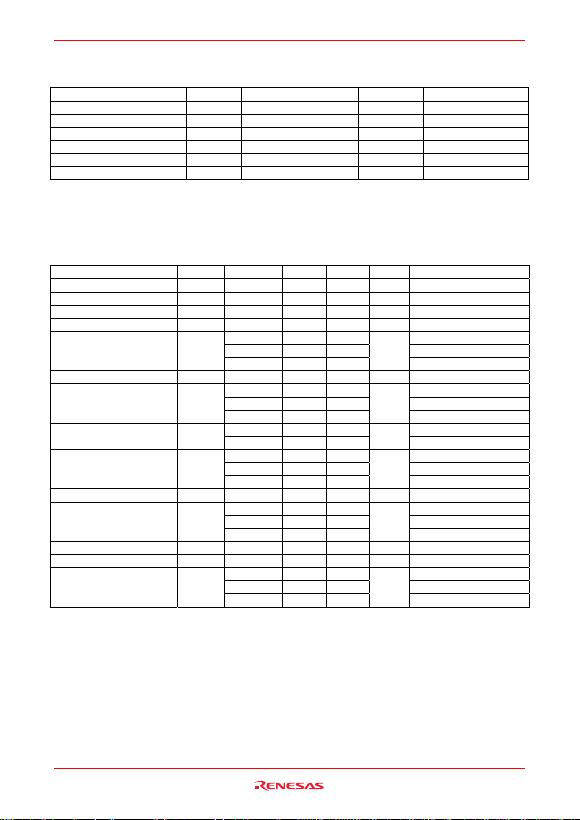HA1630D04/05/06 Series
Rev.2.00 Feb 07, 2007 page 3 of 23
Absolute Maximum Ratings
(Ta = 25°C)
Items Symbol Ratings Unit Note
Supply voltage VDD 7 V
Differential input voltage VIN(diff) –VDD to +VDD V
Input voltage VIN –0.3 to +VDD V *1
Power dissipation PT 240/145 mW TTP-8DA/MMPAK-8 *2
Operating temp. Range Topr –40 to +85 °C
Storage temp. Range Tstg –55 to +125 °C
Notes: 1. Do not apply Input Voltage exceeding VDD or 7 V.
2. The value of PTSP0008JC-B (TTP-8DAV) / PLSP0008JC-A (MMPAK-8). It computes from heat resistance
θja = 520°C/W, and 690°C/W each other.
Electrical Characteristics
(VDD = 3.0 V, Ta = 25°C)
Items Symbol Min Typ Max Unit Test Condition
Input offset voltage VIO 4.0 mV Vin = 1.5 V
Input offset current IIO (1.0) pA Vin = 1.5 V
Input bias current IIB (1.0) pA Vin = 1.5 V
Output high voltage VOH 2.9 V RL = 100 kΩ
100 200 VOH = 2.5 V (HA1630D04)
200 400 VOH = 2.5 V (HA1630D05)
Output source current IO SOURCE
400 800
μA
VOH = 2.5 V (HA1630D06)
Output low voltage VOL0.1 V RL = 100 kΩ
— (5.0) VOL = 0.5 V (HA1630D04)
— (6.0) VOL = 0.5 V (HA1630D05)
Output sink current IO SINK
— (6.5)
mA
VOL = 0.5 V (HA1630D06)
–0.05 to 2.1 (HA1630D04, HA1630D05)
Common mode input voltage
range VCM 0 to 1.9 V (HA1630D06)
— (2.0) CL = 20 pF (HA1630D04)
— (4.0) CL = 20 pF (HA1630D05)
Slew rate SR
— (8.0)
V/μs
CL = 20 pF (HA1630D06)
Voltage gain AV 60 90 dB
— (2100) CL = 20 pF (HA1630D04)
— (3300) CL = 20 pF (HA1630D05)
Gain bandwidth product BW
— (3600)
kHz
CL = 20 pF (HA1630D06)
Power supply rejection ratio PSRR 50 70 dB
Common mode rejection ratio CMRR 50 70 dB
— 400 800 RL = (HA1630D04)
— 800 1600 RL = (HA1630D05)
Supply current IDD
— 1600 3400
μA
RL = (HA1630D06)
Notes: 1. In the case of continuous current flow, use a sink current of under 4 mA.
2. ( ) : Design specification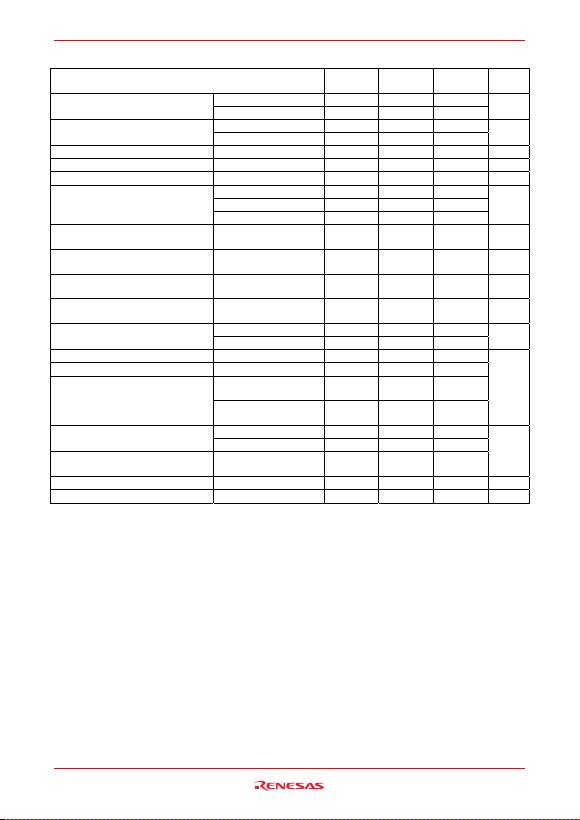HA1630D04/05/06 Series
Rev.2.00 Feb 07, 2007 page 4 of 23
Table of Graphs
Electrical Characteristics HA1630D04
Figure HA1630D05
Figure HA1630D06
Figure Test
Circuit
vs Supply voltage 1-1 2-1 3-1 Supply current IDD vs Ambient temperature 1-2 2-2 3-2 2
vs Output source current 1-3 2-3 3-3 Output high voltage VOH vs Supply voltage 1-4 2-4 3-4 4
Output source current IO SOURCE vs Ambient temperature 1-5 2-5 3-5 6
Output low voltage VOL vs Output sink current 1-6 2-6 3-6 5
Output sink current IO SINK vs Ambient temperature 1-7 2-7 3-7 6
Distribution 1-8 2-8 3-8
vs Supply voltage 1-9 2-9 3-9
Input offset voltage VIO
vs Ambient temperature 1-10 2-10 3-10
1
Common mode input
voltage range VCM vs Ambient temperature 1-11 2-11 3-11 7
Power supply rejection
ratio PSRR vs Frequency 1-12 2-12 3-12 1
Common mode rejection
ratio CMRR vs Frequency 1-13 2-13 3-13 7
Voltage gain & phase
angle AV vs Frequency 1-14 2-14 3-14 10
vs Ambient temperature 1-15 2-15 3-15 Input bias current IIB vs Input voltage 1-16 2-16 3-16 3
Slew Rate (rising) SRr vs Ambient temperature 1-17 2-17 3-17
Slew Rate (falling) SRf vs Ambient temperature 1-18 2-18 3-18
Large signal transient
response 1-19 2-19 3-19
Slew rate
Small signal transient
response 1-20 2-20 3-20
9
(0 dB) vs. Output voltage p-p 1-21 2-21 3-21
Total harmonic distortion +
noise (40 dB) vs. Output voltage p-p 1-22 2-22 3-22
Maximum p-p output
voltage vs Frequency 1-23 2-23 3-23
8
Voltage noise density vs Frequency 1-24 2-24 3-24
Channel separation vs Frequency 1-25 2-25 3-25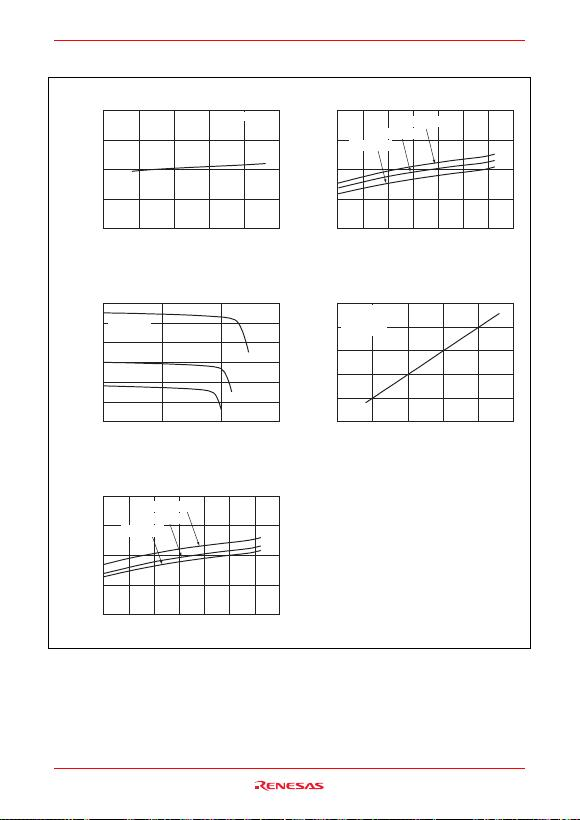HA1630D04/05/06 Series
Rev.2.00 Feb 07, 2007 page 5 of 23
Main Characteristics (HA1630D04)
Figure 1-1. HA1630D04
Supply Current vs. Supply Voltage
0
100
200
300
400
123456
Supply Voltage V
DD
(V)
Supply Current I
DD
(μA)
Figure 1-3. HA1630D04
Output High Voltage vs. Output Source Current
0
1
2
3
4
5
6
0 100 200 300
Output Source Current I
OSOURCE
(μA)
Output High Voltage V
OH
(V)
V
DD
= 5.5 V
V
DD
= 3.0 V
V
DD
= 1.8 V
Ta = 25°C
Ta = 25°C
V
DD
= 5.5 V
V
DD
= 3.0 V
V
DD
= 1.8 V
Figure 1-5. HA1630D04
Output Source Current vs. Ambient Temperature
0
100
200
300
400
40 20 0 20 40 60 80 100
Ambient Temperature Ta (°C)
Output Source Current
I
OSOURCE
(μA)
Figure 1-2. HA1630D04
Supply Current vs. Ambient Temperature
0
100
200
300
400
40 20 0 20 40 60 80 100
Ambient Temperature Ta (°C)
Supply Current I
DD
(μA)
V
DD
= 5.5 V
V
DD
= 3.0 V
V
DD
= 1.8 V
Ta = 25°C
V
DD
= 3.0 V
R
L
= 100 k
Ω
Figure 1-4. HA1630D04
Output High Voltage vs. Supply Voltage
1
2
3
4
5
6
123456
Supply Voltage V
DD
(V)
Output High Voltage V
OH
(V)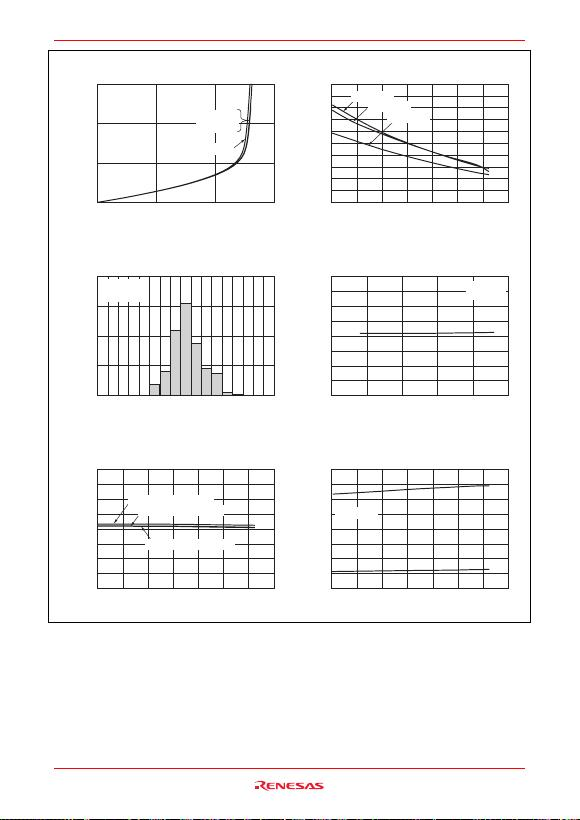HA1630D04/05/06 Series
Rev.2.00 Feb 07, 2007 page 6 of 23
Figure 1-6. HA1630D04
Output Low Voltage vs. Output Sink Current
0
0.5
1.0
1.5
0246
Output Sink Current I
OSINK
(mA)
Output Low Voltage V
OL
(V)
V
DD
= 5.5 V
V
DD
= 3.0 V
V
DD
= 3.0 V
V
DD
= 1.8 V
Figure 1-8. HA1630D04
Input Offset Voltage Distribution
0
10
20
30
40
43210 1 2 3 4
Input Offset Voltage V
IO
(mV)
Percentage (%)
Figure 1-10. HA1630D04
Input Offset Voltage vs. Ambient Temperature
4
3
2
1
0
1
2
3
4
40 20 0 20 40 60 80 100
Ambient Temperature Ta (°C)
Input Offset Voltage V
IO
(mV)
V
DD
= 5.5 V, V
IN
= 2.75 V
V
DD
= 1.8 V, V
IN
= 0.9 V
V
DD
= 3.0 V, V
IN
= 1.5 V
Figure 1-7. HA1630D04
Output Sink Current vs. Ambient Temperature
0
4
2
6
8
10
40 20 0 20 40 60 80 100
Ambient Temperature Ta (°C)
Output Sink Current
I
OSINK
(mA)
V
DD
= 5.5 V
V
DD
= 3.0 V
V
DD
= 1.8 V
Figure 1-9. HA1630D04
Input Offset Voltage vs. Supply Voltage
4
3
2
1
0
1
2
3
4
123456
Supply Voltage V
DD
(V)
Input Offset Voltage V
IO
(mV)
Ta = 25°C
V
IN
= 0.5 V
Figure 1-11. HA1630D04
Common Mode Input Voltage vs.
Ambient Temperature
1.0
0
1.0
2.0
3.0
40 20 0 20 40 60 80 100
Ambient Temperature Ta (°C)
Common Mode
Input Voltage V
CM
(V)
Ta = 25°C
V
DD
= 3.0 V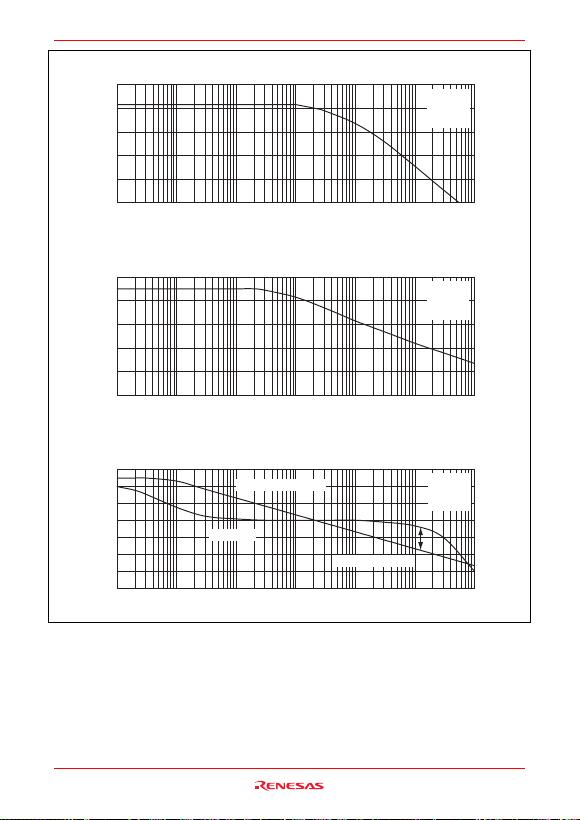HA1630D04/05/06 Series
Rev.2.00 Feb 07, 2007 page 7 of 23
Figure 1-14. HA1630D04
Open Loop Voltage Gain and Phase Angle vs. Frequency
40
20
0
20
40
60
80
100
Frequency f (Hz)
Open Loop Voltage Gain
AVOL (dB)
90
45
0
45
90
135
180
225
Phase Angle (deg)
Open Loop Voltage Gain
Phase Angle
Phase Margin: 57 deg
Figure 1-13. HA1630D04
Common Mode Rejection Ratio vs. Frequency
0
20
40
60
80
100
Frequency f (Hz)
Common Mode Rejection Ratio
CMRR (dB)
Figure 1-12. HA1630D04
Power Supply Rejection Ratio vs. Frequency
0
20
40
60
80
100
10 100 1k 10k 100k 1M 10M
10 100 1k 10k 100k 1M 10M
10 100 1k 10k 100k 1M 10M
Frequency f (Hz)
Power Supply Rejection Ratio
PSRR (dB)
Ta = 25°C
VDD = 3.0 V
RL = 1 MΩ
CL = 20 pF
Ta = 25°C
VDD = 3.0 V
RL = 1 MΩ
CL = 20 pF
Ta = 25°C
VDD = 3.0 V
RL = 1 MΩ
CL = 20 pF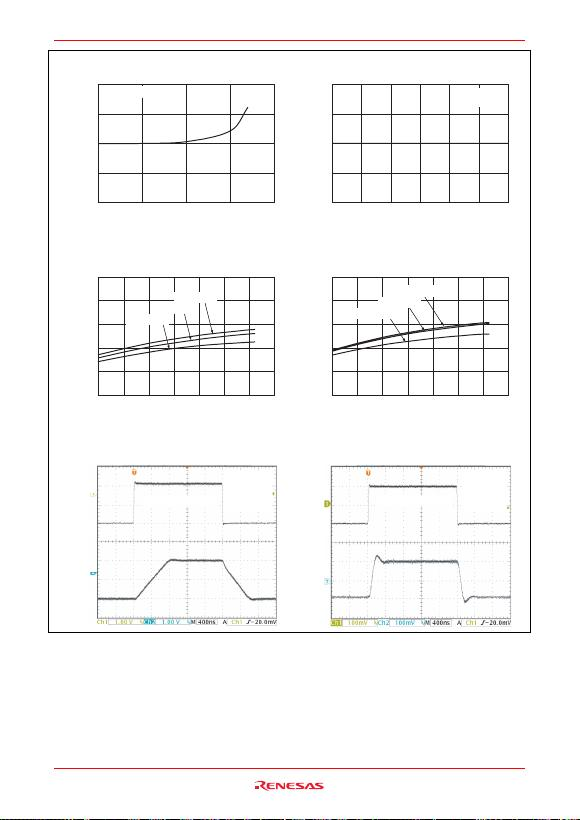HA1630D04/05/06 Series
Rev.2.00 Feb 07, 2007 page 8 of 23
1.4 V
1.6 V
1.6 V
1.4 V
0 V
2.0 V
2.0 V
0 V
Figure 1-15. HA1630D04
Input Bias Current vs. Ambient Temperature
200
100
0
100
200
0255075100
Ambient Temperature Ta (°C)
Input Bias Current I
IB
(pA)
Figure 1-16. HA1630D04
Input Bias Current vs. Input Voltage
200
100
0
100
200
0 0.5 1.0 1.5 2.0 2.5 3.0
Input Voltage V
IN
(V)
Input Bias Current I
IB
(pA)
Figure 1-20. HA1630D04
Small Signal Transient Response
Figure 1-19. HA1630D04
Large Signal Transient Response
Figure 1-17. HA1630D04
Slew Rate (rising) vs. Ambient Temperature
0
2
1
3
4
5
40 20 0 20 40 60 80 100
Ambient Temperature Ta (°C)
Slew Rate SRr (V/μs)
Figure 1-18. HA1630D04
Slew Rate (falling) vs. Ambient Temperature
0
2
1
3
4
5
40 20 0 20 40 60 80 100
Ambient Temperature Ta (°C)
Slew Rate SRf (V/μs)
V
DD
= 5.5 V
V
DD
= 3.0 V
V
DD
= 1.8 V
V
DD
= 5.5 V
V
DD
= 3.0 V
V
DD
= 1.8 V
Ta = 25°C
V
DD
= 3.0 V
R
L
= 100 kΩ
C
L
= 20 pF
Ta = 25°C
V
DD
= 3.0 V
R
L
= 100 kΩ
C
L
= 20 pF
V
DD
= 3.0 V Ta = 25°C
V
DD
= 3.0 V
Vin = 2.1 Vp-p, 250 kHz Vin = 0.2 Vp-p, 250 kHz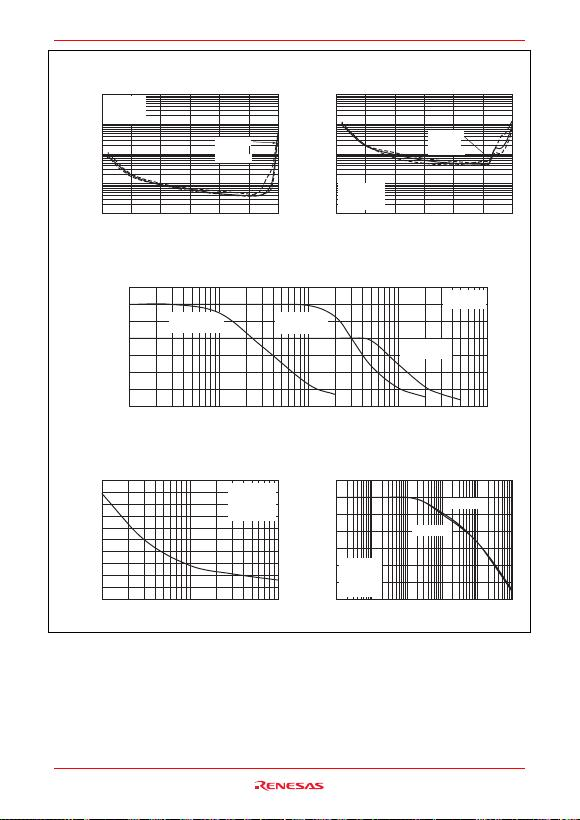HA1630D04/05/06 Series
Rev.2.00 Feb 07, 2007 page 9 of 23
Figure 1-21. HA1630D04
Total Harmonic Distortion + Noise vs.
Output Voltage p-p
0.001
0.01
0.1
1
10
0 0.5 1.0 1.5 2.0 2.5 3.0
Output Voltage Vout p-p (V)
T.H.D. + Noise (%)
V
DD
= 3.0 V
Ta = 25°C
Gain = 0 dB
f = 10 kHz
f = 100 Hz
f = 1 kHz
V
DD
= 3.0 V
Ta = 25°C
Gain = 40 dB
f = 10 kHz
f = 100 Hz
f = 1 kHz
Figure 1-22. HA1630D04
Total Harmonic Distortion + Noise vs.
Output Voltage p-p
0.001
0.01
0.1
1
10
0 0.5 1.0 1.5 2.0 2.5 3.0
Output Voltage Vout p-p (V)
T.H.D. + Noise (%)
Figure 1-24. HA1630D04
Voltage Noise Density vs. Frequency
0
80
40
120
160
200
100 1k 10k
Frequency f (Hz)
Voltage Noise Density
(nVms/Hz)
Figure 1-25. HA1630D04
Channel Separation vs. Frequency
0
60
40
20
100
80
120
140
100 1k 10k 100k 1M 10M
Frequency f (Hz)
Channel Separation C.S (dB)
Figure 1-23. HA1630D04
Voltage Output p-p vs. Frequency
0
0.5
1.0
1.5
2.0
2.5
3.0
3.5
1k 10k 100k 1M 10M
Frequency f (Hz)
Voltage Output Vout p-p (V)
Gain = 40 dB,
V
IN
= 0.03 Vp-p
Gain = 20 dB,
V
IN
= 0.3 Vp-p
Gain = 0 dB,
V
IN
= 2.0 Vp-p
Ta = 25°C
V
DD
= 3.0 V
CH2CH1
CH1CH2
V
DD
= 3.0 V
Ta = 25°C
Gain = 40 dB
R
S
= 1 kΩ
V
DD
= 3.0 V
Ta = 25°C
R
L
= 1 MΩ
C
L
= 20 pF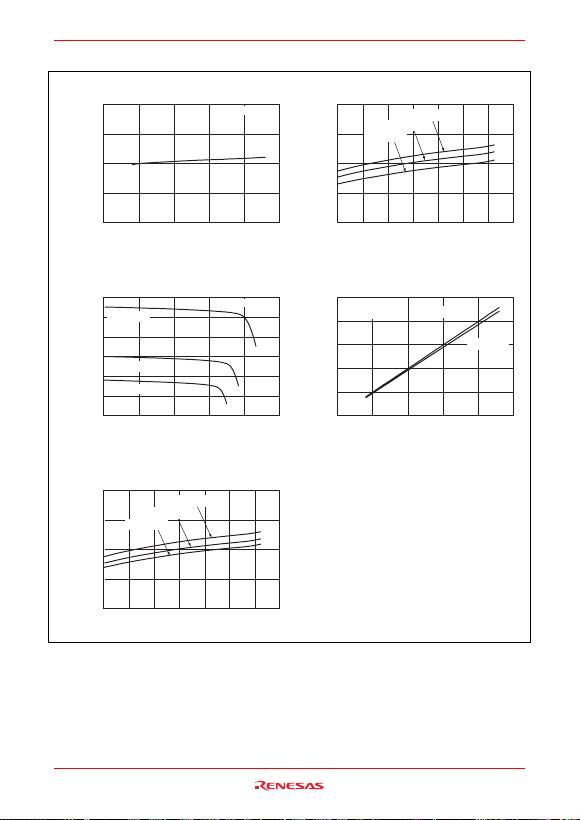HA1630D04/05/06 Series
Rev.2.00 Feb 07, 2007 page 10 of 23
Main Characteristics (HA1630D05)
Figure 2-1. HA1630D05
Supply Current vs. Supply Voltage
0
200
400
600
800
123456
Supply Voltage V
DD
(V)
Supply Current I
DD
(μA)
Figure 2-3. HA1630D05
Output High Voltage vs. Output Source Current
0
1
2
3
4
5
6
0 100 200 300 400 500
Output Source Current I
OSOURCE
(μA)
Output High Voltage V
OH
(V)
V
DD
= 5.5 V
V
DD
= 3.0 V
V
DD
= 1.8 V
Ta = 25°C
Ta = 25°C
V
DD
= 5.5 V
V
DD
= 3.0 V
V
DD
= 1.8 V
Figure 2-5. HA1630D05
Output Source Current vs. Ambient Temperature
0
200
400
600
800
40 20 0 20 40 60 80 100
Ambient Temperature Ta (°C)
Output Source Current
I
OSOURCE
(μA)
Figure 2-2. HA1630D05
Supply Current vs. Ambient Temperature
0
200
400
600
800
40 20 0 20 40 60 80 100
Ambient Temperature Ta (°C)
Supply Current I
DD
(μA)
V
DD
= 5.5 V
V
DD
= 3.0 V
V
DD
= 1.8 V
R
L
= 20 k
Ω
R
L
= 100 k
Ω
Ta = 25°C
V
DD
= 3.0 V
Figure 2-4. HA1630D05
Output High Voltage vs. Supply Voltage
1
2
3
4
5
6
123456
Supply Voltage V
DD
(V)
Output High Voltage V
OH
(V)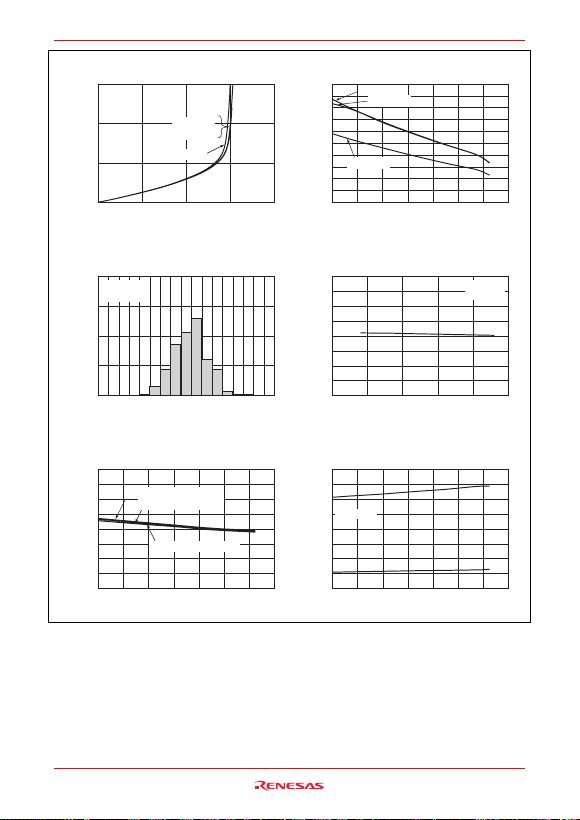HA1630D04/05/06 Series
Rev.2.00 Feb 07, 2007 page 11 of 23
Figure 2-6. HA1630D05
Output Low Voltage vs. Output Sink Current
0
0.5
1.0
1.5
04628
Output Sink Current IOSINK (mA)
Output Low Voltage VOL (V)
VDD = 5.5 V
VDD = 3.0 V
VDD = 1.8 V
Figure 2-8. HA1630D05
Input Offset Voltage Distribution
0
10
20
30
40
43210 1 2 3 4
Input Offset Voltage VIO (mV)
Percentage (%)
VDD = 3.0 V
Figure 2-10. HA1630D05
Input Offset Voltage vs. Ambient Temperature
4
3
2
1
0
1
2
3
4
40 20 0 20 40 60 80 100
Ambient Temperature Ta (°C)
Input Offset Voltage VIO (mV)
VDD = 5.5 V, VIN = 2.75 V
VDD = 1.8 V, VIN = 0.5 V
VDD = 3.0 V, VIN = 1.5 V
Figure 2-7. HA1630D05
Output Sink Current vs. Ambient Temperature
0
4
2
6
8
10
40 20 0 20 40 60 80 100
Ambient Temperature Ta (°C)
Output Sink Current
IOSINK (mA)
VDD = 5.5 V
VDD = 3.0 V
VDD = 1.8 V
Figure 2-9. HA1630D05
Input Offset Voltage vs. Supply Voltage
4
3
2
1
0
1
2
3
4
123456
Supply Voltage VDD (V)
Input Offset Voltage VIO (mV)
Ta = 25°C
VIN = 0.5 V
Figure 2-11. HA1630D05
Common Mode Input Voltage vs.
Ambient Temperature
1.0
0
1.0
2.0
3.0
40 20 0 20 40 60 80 100
Ambient Temperature Ta (°C)
Common Mode
Input Voltage VCM (V)
Ta = 25°C
VDD = 3.0 V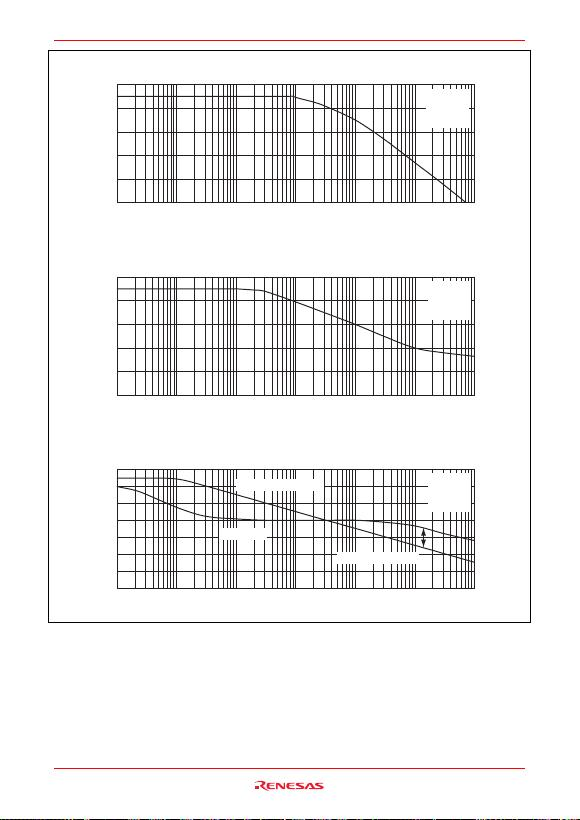HA1630D04/05/06 Series
Rev.2.00 Feb 07, 2007 page 12 of 23
Figure 2-14. HA1630D05
Open Loop Voltage Gain and Phase Angle vs. Frequency
40
20
0
20
40
60
80
100
Frequency f (Hz)
Open Loop Voltage Gain
AVOL (dB)
90
45
0
45
90
135
180
225
Phase Angle (deg)
Open Loop Voltage Gain
Phase Angle
Figure 2-13. HA1630D05
Common Mode Rejection Ratio vs. Frequency
0
20
40
60
80
100
Frequency f (Hz)
Common Mode Rejection Ratio
CMRR (dB)
Figure 2-12. HA1630D05
Power Supply Rejection Ratio vs. Frequency
0
20
40
60
80
100
10 100 1k 10k 100k 10M
10 100 1k 10k 100k
1M
1M 10M
10 100 1k 10k 100k 1M 10M
Frequency f (Hz)
Power Supply Rejection Ratio
PSRR (dB)
Ta = 25°C
VDD = 3.0 V
RL = 1 MΩ
CL = 20 pF
Ta = 25°C
VDD = 3.0 V
RL = 1 MΩ
CL = 20 pF
Ta = 25°C
VDD = 3.0 V
RL = 1 MΩ
CL = 20 pF
Phase Margin: 55 deg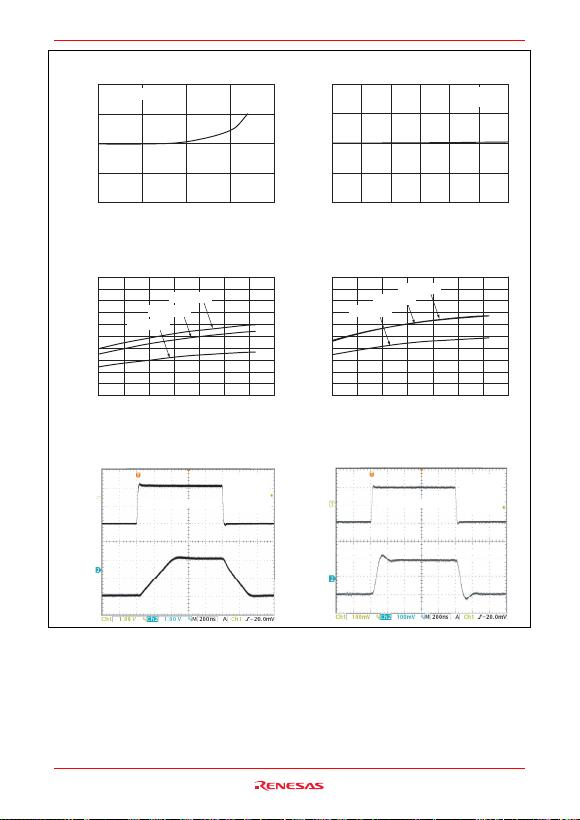HA1630D04/05/06 Series
Rev.2.00 Feb 07, 2007 page 13 of 23
Figure 2-15. HA1630D05
Input Bias Current vs. Ambient Temperature
200
100
0
100
200
0 25 50 75 100
Ambient Temperature Ta (°C)
Input Bias Current IIB (pA)
Figure 2-16. HA1630D05
Input Bias Current vs. Input Voltage
200
100
0
100
200
0 0.5 1.0 1.5 2.0 2.5 3.0
Input Voltage VIN (V)
Input Bias Current IIB (pA)
Figure 2-20. HA1630D05
Small Signal Transient Response
Figure 2-19. HA1630D05
Large Signal Transient Response
Figure 2-17. HA1630D05
Slew Rate (rising) vs. Ambient Temperature
0
4
2
6
8
10
40 20 0 20 40 60 80 100
Ambient Temperature Ta (°C)
Slew Rate SRr (V/μs)
Figure 2-18. HA1630D05
Slew Rate (falling) vs. Ambient Temperature
0
4
2
6
8
10
40 20 0 20 40 60 80 100
Ambient Temperature Ta (°C)
Slew Rate SRf (V/μs)
VDD = 5.5 V
VDD = 3.0 V
VDD = 1.8 V
VDD = 5.5 V
VDD = 3.0 V
VDD = 1.8 V
Ta = 25°C
VDD = 3.0 V
VDD = 3.0 V
1.4 V
1.6 V
1.6 V
1.4 V
0V
2.0 V
2.0 V
0 V
Ta = 25°C
VDD = 3.0 V
RL = 100 kΩ
CL = 20 pF
Ta = 25°C
VDD = 3.0 V
RL = 100 kΩ
CL = 20 pF
V
IN
= 2.1 V
p-p
, 500 kHz V
IN
= 0.2 V
p-p
, 500 kHz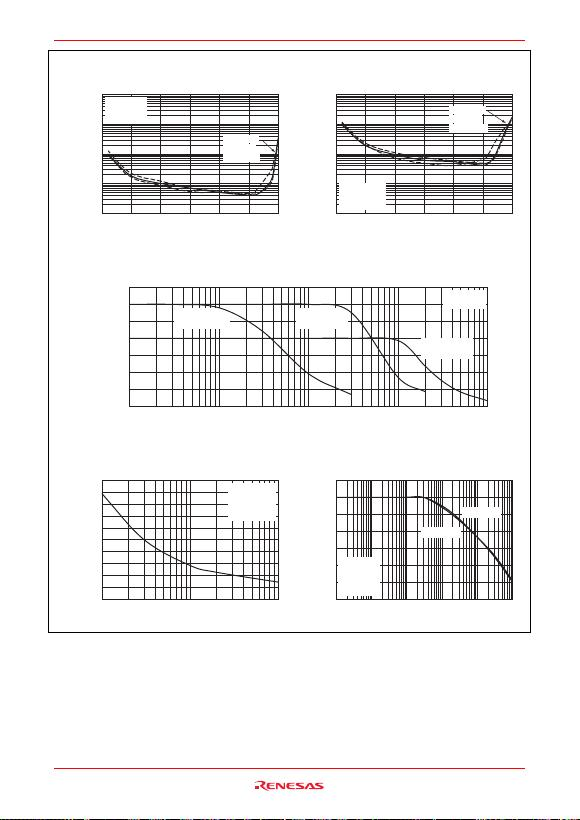HA1630D04/05/06 Series
Rev.2.00 Feb 07, 2007 page 14 of 23
Figure 2-21. HA1630D05
Total Harmonic Distortion + Noise vs.
Output Voltage p-p
0.001
0.01
0.1
1
10
0 0.5 1.0 1.5 2.0 2.5 3.0
Output Voltage Vout p-p (V)
T.H.D. + Noise (%)
V
DD
= 3.0 V
Ta = 25°C
Gain = 0 dB
f = 10 kHz
f = 100 Hz
f = 1 kHz
V
DD
= 3.0 V
Ta = 25°C
Gain = 40 dB
f = 10 kHz
f = 100 Hz
f = 1 kHz
Figure 2-22. HA1630D05
Total Harmonic Distortion + Noise vs.
Output Voltage p-p
0.001
0.01
0.1
1
10
0 0.5 1.0 1.5 2.0 2.5 3.0
Output Voltage Vout p-p (V)
T.H.D. + Noise (%)
Figure 2-24. HA1630D05
Voltage Noise Density vs. Frequency
0
80
40
120
160
200
100 1k 10k
Frequency f (Hz)
Voltage Noise Density
(nVms/Hz)
Figure 2-25. HA1630D05
Channel Separation vs. Frequency
0
60
40
20
100
80
120
140
100 1k 10k 100k 1M 10M
Frequency f (Hz)
Channel Separation C.S (dB)
Figure 2-23. HA1630D05
Voltage Output p-p vs. Frequency
0
0.5
1.0
1.5
2.0
2.5
3.0
3.5
1k 10k 100k 1M 10M
Frequency f (Hz)
Voltage Output Vout p-p (V)
Gain = 40 dB,
V
IN
= 0.03 Vp-p
Gain = 20 dB,
V
IN
= 0.3 Vp-p
Gain = 0 dB,
V
IN
= 2.0 Vp-p
Ta = 25°C
V
DD
= 3.0 V
CH2CH1
CH1CH2
V
DD
= 3.0 V
Ta = 25°C
Gain = 40 dB
R
S
= 1 kΩ
V
DD
= 3.0 V
Ta = 25°C
R
L
= 1 MΩ
C
L
= 20 pF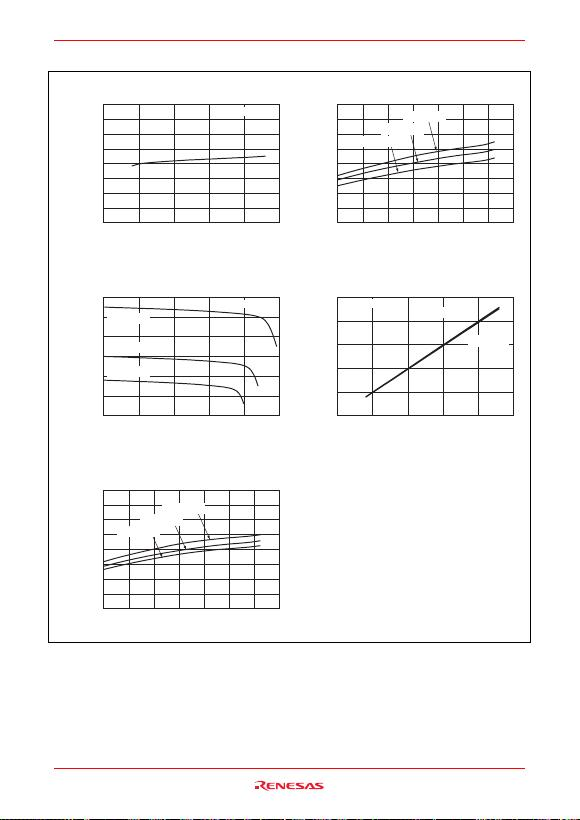HA1630D04/05/06 Series
Rev.2.00 Feb 07, 2007 page 15 of 23
Main Characteristics (HA1630D06)
Figure 3-1. HA1630D06
Supply Current vs. Supply Voltage
0
400
800
1200
1600
123456
Supply Voltage V
DD
(V)
Supply Current I
DD
(μA)
Figure 3-3. HA1630D06
Output High Voltage vs. Output Source Current
0
1
2
3
4
5
6
0400200 600 800 1000
Output Source Current I
OSOURCE
(μA)
Output High Voltage V
OH
(V)
V
DD
= 5.5 V
V
DD
= 3.0 V
V
DD
= 1.8 V
Ta = 25°C
Ta = 25°C
V
DD
= 5.5 V
V
DD
= 3.0 V
V
DD
= 1.8 V